# Titanic: Machine Learning from Disaster¶

## Contents of the Notebook¶

### Part2 : Load and check data¶

1) load data

2) Feature type

3) Outlier detection

4) Missing values



### Part3 : Feature analysis¶

1) Numerical values

2) Categorical values



### Part4 : Filling missing Values¶

1) Age



### Part5 : Feature engineering¶

1) Name/Title

2) Family Size

3) Cabin

4) Ticket



### Part6 : Modeling¶

1) Prepare input and test data

2) Model Performance

3) Model choice and submission

## 1. Introduction¶

I choosed the Titanic competition which is a good way to introduce feature engineering and ensemble modeling.

This script follows three main parts:

• Feature analysis
• Feature engineering
• Modeling
In :
import pandas as pd
import numpy as np
import matplotlib.pyplot as plt
import seaborn as sns
%matplotlib inline

from scipy import stats
from collections import Counter

import warnings
warnings.filterwarnings('ignore')

sns.set(style='white', context='notebook', palette='deep')


## 2. Load and check data¶

In :
# Load train and Test set
IDtest = test["PassengerId"]

In :
# Check the data set
print("Train data : ", train.shape)
print("Test  data : ", test.shape)

Train data :  (891, 12)
Test  data :  (418, 11)

In :
# Check the train data set's columns
print("Train data columns Qty :", len(train.columns), "\n\n")
print("Train data columns :", train.columns)

Train data columns Qty : 12

Train data columns : Index(['PassengerId', 'Survived', 'Pclass', 'Name', 'Sex', 'Age', 'SibSp',
'Parch', 'Ticket', 'Fare', 'Cabin', 'Embarked'],
dtype='object')

In :
# states of train data set
# describe the train
train.describe()

Out:
PassengerId Survived Pclass Age SibSp Parch Fare
count 891.000000 891.000000 891.000000 714.000000 891.000000 891.000000 891.000000
mean 446.000000 0.383838 2.308642 29.699118 0.523008 0.381594 32.204208
std 257.353842 0.486592 0.836071 14.526497 1.102743 0.806057 49.693429
min 1.000000 0.000000 1.000000 0.420000 0.000000 0.000000 0.000000
25% 223.500000 0.000000 2.000000 20.125000 0.000000 0.000000 7.910400
50% 446.000000 0.000000 3.000000 28.000000 0.000000 0.000000 14.454200
75% 668.500000 1.000000 3.000000 38.000000 1.000000 0.000000 31.000000
max 891.000000 1.000000 3.000000 80.000000 8.000000 6.000000 512.329200
In :
# states of train data set
# describe the data by dtype

summary_train = pd.DataFrame()
for col in train.columns:

# column's name
column_name = col

# check column's type
dtype = train[column_name].dtype

# check the qty of not null data per each column
actual_value_qty = len(train.loc[train[column_name].notnull()])

# length of each columns
rows = len(train[column_name])

# percent of not null values per columns
actual_value_percent = round((actual_value_qty / rows)*100,1)

# count the unique values per columns
unique_values = len(train[column_name].unique())

# sum up the null values per columns
null_qty = train[column_name].isnull().sum()

# make the dataframe
data = {'column_name' : column_name, 'dtype' : dtype, 'actual_value_qty' : actual_value_qty,'null_qty' : null_qty, \
'actual_value_percent(%)' : actual_value_percent  ,'unique_values_qty' : unique_values}

summary_train = summary_train.append(data, ignore_index = True)

summary_train.pivot_table(index = ['dtype', 'column_name'])

Out:
actual_value_percent(%) actual_value_qty null_qty unique_values_qty
dtype column_name
int64 Parch 100.0 891.0 0.0 7.0
PassengerId 100.0 891.0 0.0 891.0
Pclass 100.0 891.0 0.0 3.0
SibSp 100.0 891.0 0.0 7.0
Survived 100.0 891.0 0.0 2.0
float64 Age 80.1 714.0 177.0 89.0
Fare 100.0 891.0 0.0 248.0
object Cabin 22.9 204.0 687.0 148.0
Embarked 99.8 889.0 2.0 4.0
Name 100.0 891.0 0.0 891.0
Sex 100.0 891.0 0.0 2.0
Ticket 100.0 891.0 0.0 681.0

- Age, Cabin and Embarked on train data have some missing values. Escpecially, Cabin columns have lot of missing values

### 2-2 Feature type¶

1) Seperate Numerical feature and Categorical feature 
In :
# Since Pclass is Categorical feature, I am going to convet it to string in both train and test set
train["Pclass"] = train["Pclass"].astype("str")
test["Pclass"] = test["Pclass"].astype("str")

In :
numerical_features = []
categorical_features = []
for f in train.columns:
if train.dtypes[f] != 'object':
numerical_features.append(f)
else:
categorical_features.append(f)

In :
print("Numerical Features Qty :", len(numerical_features),"\n")
print("Numerical Features : ", numerical_features, "\n\n")
print("Categorical Features Qty :", len(categorical_features),"\n")
print("Categorical Features :", categorical_features)

Numerical Features Qty : 6

Numerical Features :  ['PassengerId', 'Survived', 'Age', 'SibSp', 'Parch', 'Fare']

Categorical Features Qty : 6

Categorical Features : ['Pclass', 'Name', 'Sex', 'Ticket', 'Cabin', 'Embarked']


### 2.3 Outlier detection¶

1) By box-and-whisker's IQR

- The Tukey method (Tukey JW., 1977) to detect ouliers which defines an interquartile range comprised
between the 1st and 3rd quartile of the distribution values (IQR).
In :
# Outlier detection by Box plot

def detect_outliers(data, features):

outlier_indices = []
# iterate over features(columns)
for feature in features:
# 1st quartile (25%)
Q1 = np.percentile(data[feature], 25)
# 3rd quartile (75%)
Q3 = np.percentile(data[feature], 75)
# Interquartile range (IQR)
IQR = Q3 - Q1

# outlier step
outlier_step = 1.5 * IQR

# determine a list of indices of outliers for feature col
outliers = data[(data[feature] < Q1 - outlier_step) | (data[feature] > Q3 + outlier_step )].index

# append the found outlier indices for col to the list of outlier indices
outlier_indices.extend(outliers)

outlier_indices = Counter(outlier_indices)
outliers = list( num for num, qty in outlier_indices.items() if qty > 2 )

return outliers


Note : I decided to detect outliers from the numerical values features (Age, SibSp, Sarch and Fare)

In :
# detect outliers from Age, SibSp , Parch and Fare
Outliers_numerical_features = detect_outliers(train,["Age", "SibSp","Parch", "Fare"])

In :
train.loc[Outliers_numerical_features]

Out:
PassengerId Survived Pclass Name Sex Age SibSp Parch Ticket Fare Cabin Embarked
27 28 0 1 Fortune, Mr. Charles Alexander male 19.0 3 2 19950 263.00 C23 C25 C27 S
88 89 1 1 Fortune, Miss. Mabel Helen female 23.0 3 2 19950 263.00 C23 C25 C27 S
159 160 0 3 Sage, Master. Thomas Henry male NaN 8 2 CA. 2343 69.55 NaN S
180 181 0 3 Sage, Miss. Constance Gladys female NaN 8 2 CA. 2343 69.55 NaN S
201 202 0 3 Sage, Mr. Frederick male NaN 8 2 CA. 2343 69.55 NaN S
324 325 0 3 Sage, Mr. George John Jr male NaN 8 2 CA. 2343 69.55 NaN S
341 342 1 1 Fortune, Miss. Alice Elizabeth female 24.0 3 2 19950 263.00 C23 C25 C27 S
792 793 0 3 Sage, Miss. Stella Anna female NaN 8 2 CA. 2343 69.55 NaN S
846 847 0 3 Sage, Mr. Douglas Bullen male NaN 8 2 CA. 2343 69.55 NaN S
863 864 0 3 Sage, Miss. Dorothy Edith "Dolly" female NaN 8 2 CA. 2343 69.55 NaN S

- Found 10 outliers and I am going to remove it
In :
# drop outliers
train = train.drop(Outliers_numerical_features, axis = 0).reset_index(drop=True)


### 2.4 Missing values¶

1) join the train and test set
In :
# in order to handle all missing data
train_len = len(train)
all_data =  pd.concat([train, test], axis=0).reset_index(drop=True)

2) check for null and missing value
In :
# Fill empty and NaNs values with NaN
all_data = all_data.fillna(np.nan)

# Copy all_data
all_data_cp = all_data.copy()

# check for null values
all_data_null = all_data_cp.isnull().sum()
all_data_null = all_data_null.drop(all_data_null[all_data_null == 0].index).sort_values(ascending=False)

# drop the null values of Survived because Survived missing values correspond to the join testing dataset
del all_data_null['Survived']

In :
# make missing dataframe
all_data_missing = pd.DataFrame({'Missing Numbers' :all_data_null})
all_data_null =  all_data_null / len(all_data_cp)*100

# draw the graph for missing data
f, ax = plt.subplots(figsize=(15, 6))
plt.xticks(rotation='90')
sns.barplot(x=all_data_null.index, y=all_data_null)
plt.xlabel('Features', fontsize=15)
plt.ylabel('Percent of missing values', fontsize=15)
plt.title('Percent missing data by feature', fontsize=15)

print("Missing Data Features's Qty : " , all_data_missing.count().values)
print("Total Missing Data's Qty : " , all_data_missing.sum().values)

Missing Data Features's Qty :  
Total Missing Data's Qty :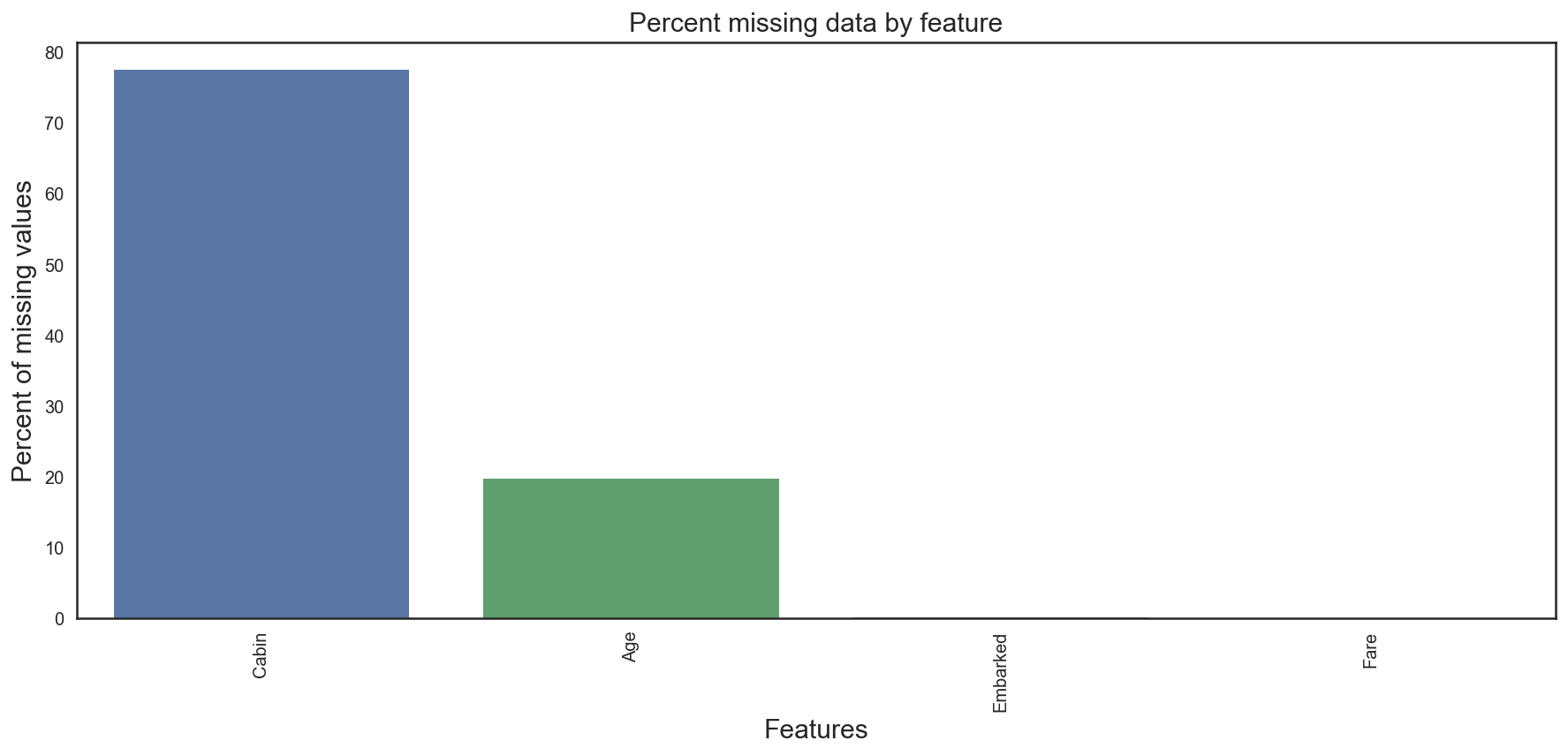- Age and Cabin features have an important part of missing values.

## 3. Feature analysis¶

### 3.1 Numerical values¶

In :
# Correlation matrix between numerical values (SibSp Parch Age and Fare values) and Survived
ls_numeric = ["Survived","SibSp","Parch","Age","Fare"]
corr = train[ls_numeric].corr()
with sns.axes_style("white"):
ax = sns.heatmap(corr, mask = mask, annot=True, fmt = ".2f", cmap = "YlGnBu")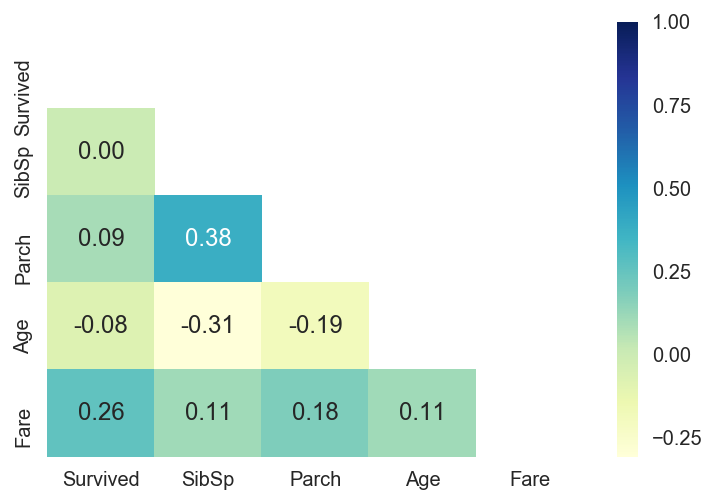- Only Fare feature seems to have a significative correlation with the survival probability.

- It doesn't mean that the other features are not usefull. Subpopulations in these features can be correlated with the survival. To determine this, we need to explore in detail these features

#### SibSP¶

Definition : Number of siblings / spouses aboard the Titanic

• Sibling = brother, sister, stepbrother, stepsister
• Spouse = husband, wife (mistresses and fiancés were ignored)
In :
# Explore SibSp feature vs Survived
g = sns.factorplot(x="SibSp",y="Survived",data=train,kind="bar", size = 5 , palette = "muted")
g.despine(left=True)
g = g.set_ylabels("survival probability")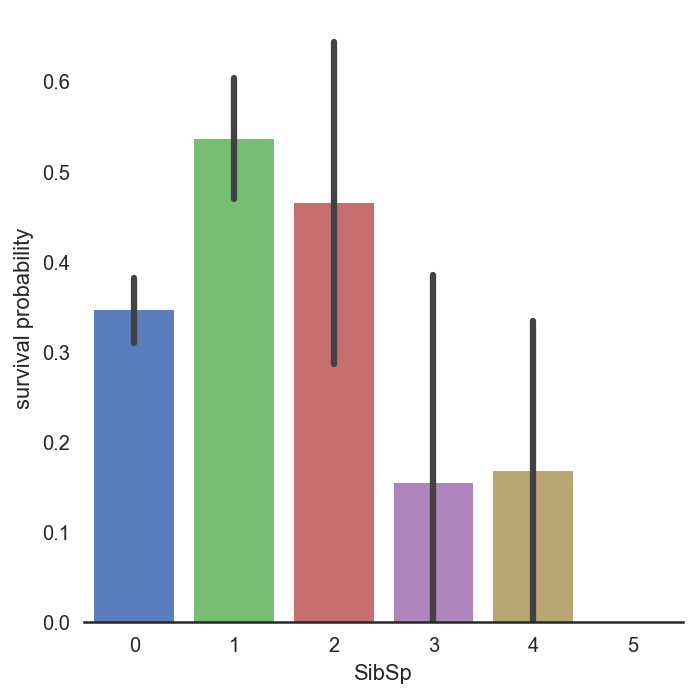- It seems that passengers having a lot of siblings/spouses have less chance to survive

- Single passengers (0 SibSP) or with two other persons (SibSP 1 or 2) have more chance to survive

#### Parch¶

Definition : Number of parents / children aboard the Titanic

• Parent = mother, father
• Child = daughter, son, stepdaughter, stepson
• Some children travelled only with a nanny, therefore parch=0 for them.
In :
# Explore Parch feature vs Survived
g  = sns.factorplot(x="Parch",y="Survived",data=train,kind="bar", size = 5 , palette = "muted")
g.despine(left=True)
g = g.set_ylabels("survival probability")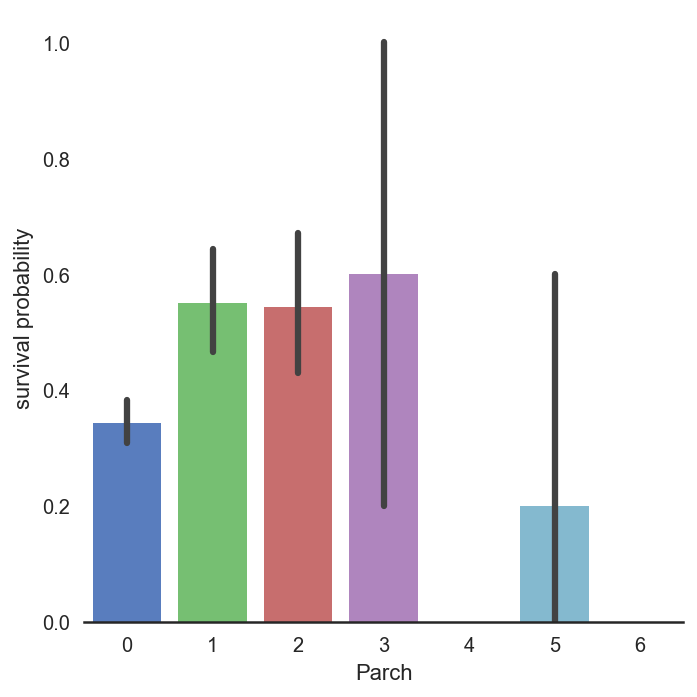- Couple and small families have more chance to survive, more than single (Parch 0),and large families (Parch 5,6 )

#### Age¶

Definition : The age of the passenger

• Age is fractional if less than 1
• If the age is estimated, is it in the form of xx.5
In :
# Explore Age vs Survived
g = sns.FacetGrid(train, col='Survived')
g = g.map(sns.distplot, "Age")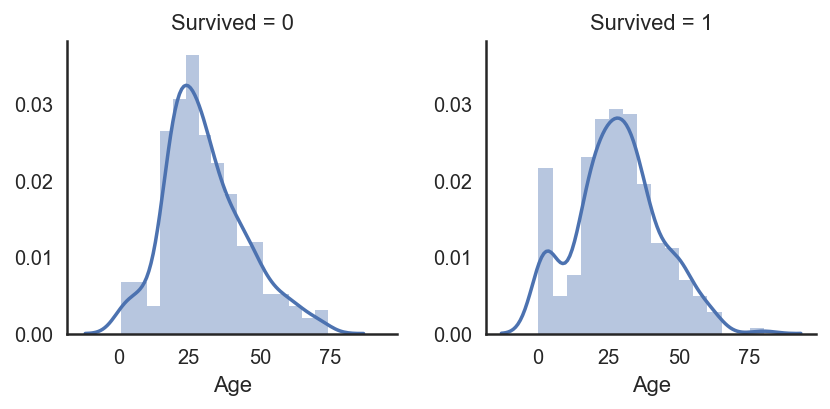- Age distribution seems to be a tailed distribution, maybe a gaussian distribution.

- Notice that age distributions are not the same in the survived and not survived subpopulations.

- There is a peak corresponding to young passengers, that have survived. We also see that passengers between 60-80 have less survived. So it seems that very young passengers have more chance to survive.
In :
# Explore Age distibution
g = sns.kdeplot(train["Age"][(train["Survived"] == 0) & (train["Age"].notnull())], color="Red", shade = True)
g = sns.kdeplot(train["Age"][(train["Survived"] == 1) & (train["Age"].notnull())], ax =g, color="Blue", shade= True)
g.set_xlabel("Age")
g.set_ylabel("Frequency")
g = g.legend(["Not Survived","Survived"])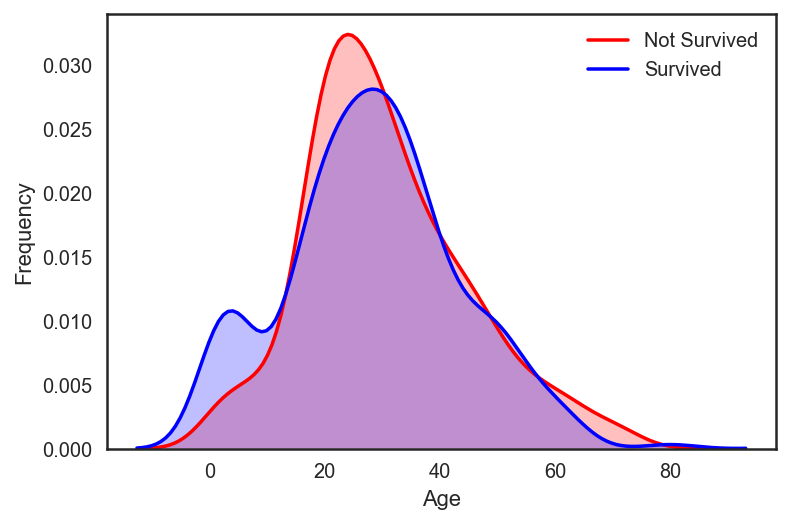- When we superimpose the two densities , we cleary see a peak correponsing (between 0 and 10) to babies and young childrens.

#### Fare¶

Definition : Passenger fare

In :
# check how many missing values on Fare
all_data["Fare"].isnull().sum()

Out:
1
In :
#Fill Fare missing values with the median value
all_data["Fare"] = all_data["Fare"].fillna(all_data["Fare"].median())


- Since we have one missing value , i decided to fill it with the median value which will not have an important effect on the prediction.
In :
# Explore Fare distribution
plt.figure(figsize=(12,5))

plt.subplot(131)
sns.distplot(all_data["Fare"])

plt.subplot(132)
stats.probplot(all_data["Fare"], plot=plt)

plt.subplot(133)
sns.boxplot(all_data["Fare"])
plt.tight_layout()
plt.show()

print("Skewness: %f" % all_data['Fare'].skew())
print("Kurtosis: %f" % all_data['Fare'].kurt())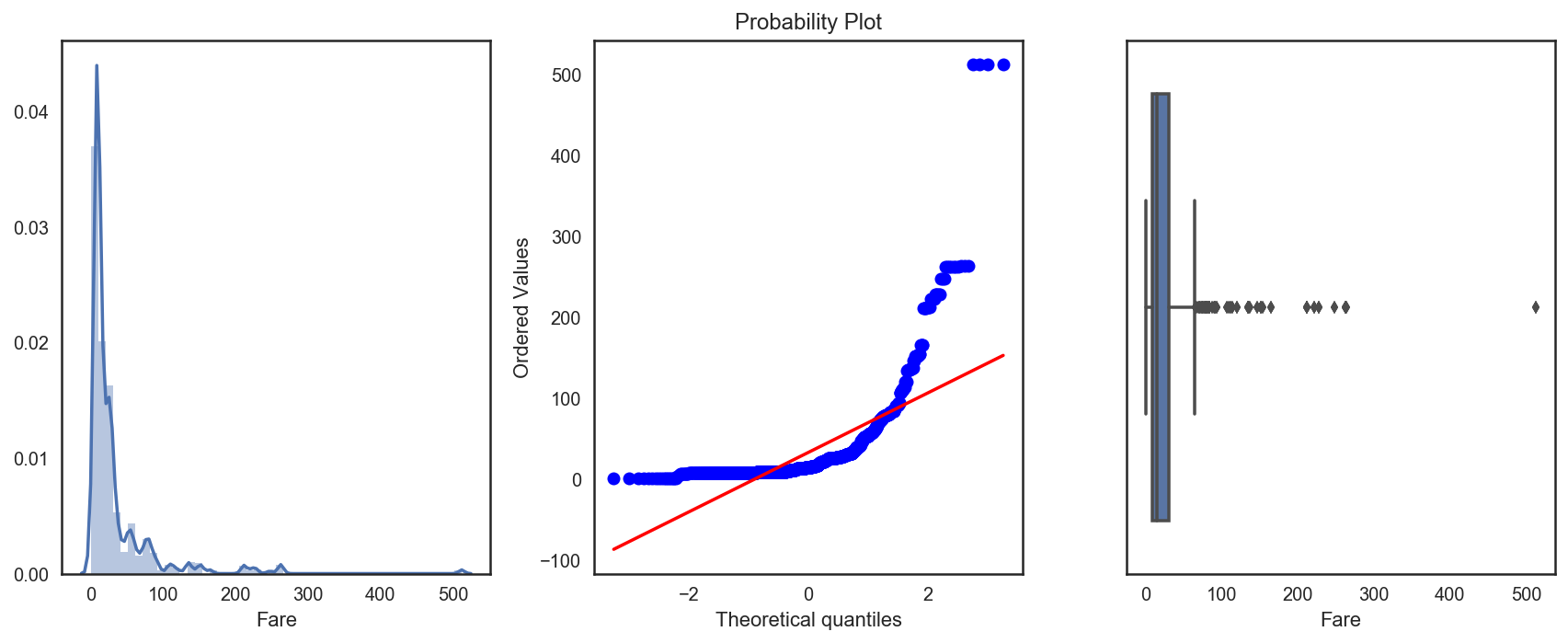Skewness: 4.511862
Kurtosis: 29.183273


- As we can see, Fare distribution is very skewed. This can lead to overweigth very high values in the model, even if it is scaled.

- In this case, it is better to transform it with the log function to reduce this skew. 
In :
all_data["Fare"] = np.log1p(all_data["Fare"])

# Explore Fare distribution
plt.figure(figsize=(12,5))

plt.subplot(131)
sns.distplot(all_data["Fare"])

plt.subplot(132)
stats.probplot(all_data["Fare"], plot=plt)

plt.subplot(133)
sns.boxplot(all_data["Fare"])
plt.tight_layout()
plt.show()

print("Skewness: %f" % all_data['Fare'].skew())
print("Kurtosis: %f" % all_data['Fare'].kurt())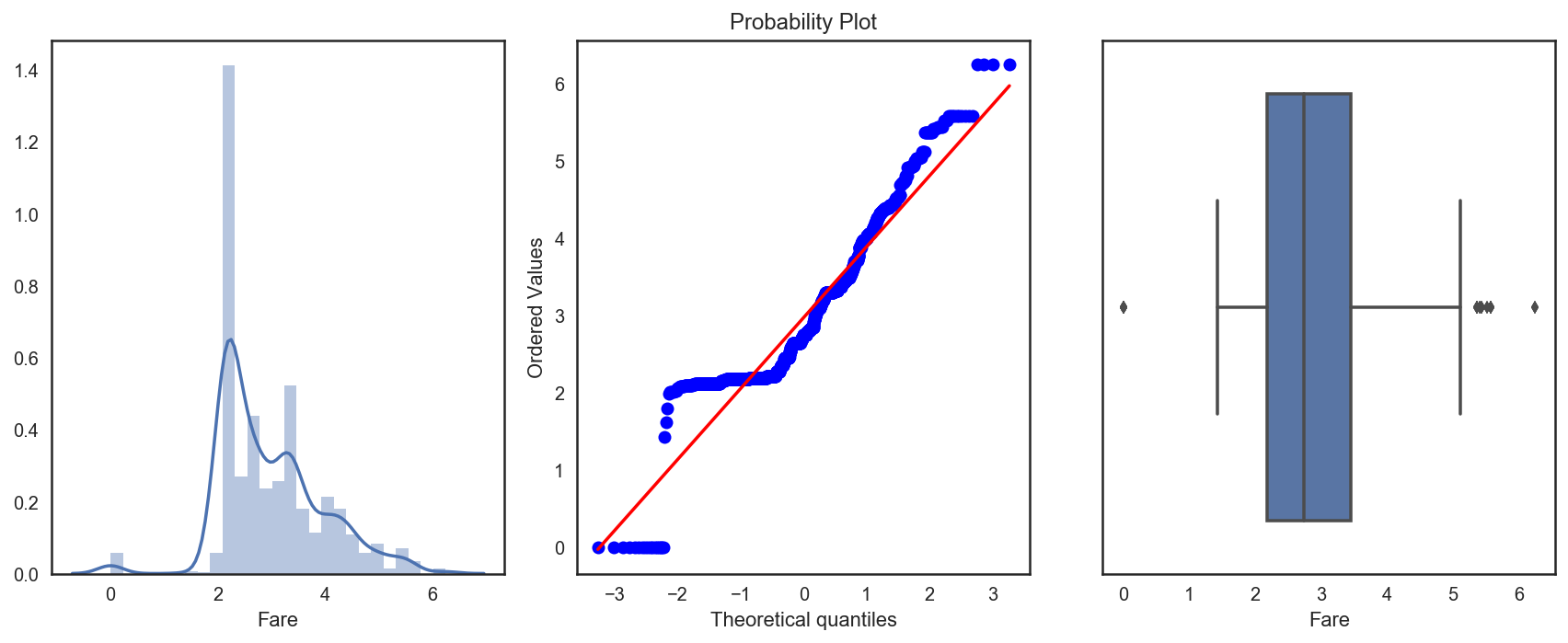Skewness: 0.544004
Kurtosis: 0.921062


- Skewness is clearly reduced after the log transformation

### 3.2 Categorical values¶

#### Sex¶

Definition : Passenger Sex

In :
g = sns.barplot(x="Sex",y="Survived",data=train)
g = g.set_ylabel("Survival Probability")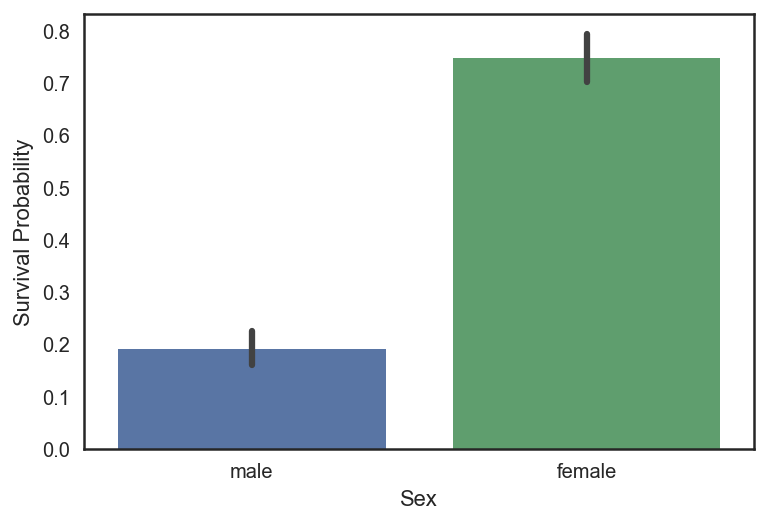- It is clearly obvious that Male have less chance to survive than Female.

- Sex, might play an important role in the prediction of the survival.

#### Pclass¶

Definition : A proxy for socio-economic status (SES)

• 1st = Upper
• 2nd = Middle
• 3rd = Lower
In :
# Explore Pclass vs Survived
plt.figure(figsize=(15,6))

ax1 = plt.subplot(1,2,1)
sns.barplot(x="Pclass",y="Survived",data=train, palette = "muted", ax=ax1)

# Explore Pclass vs Survived by Sex
ax2 = plt.subplot(1,2,2)
sns.barplot(x="Pclass", y="Survived", hue="Sex", data=train, palette="muted", ax=ax2)

plt.show()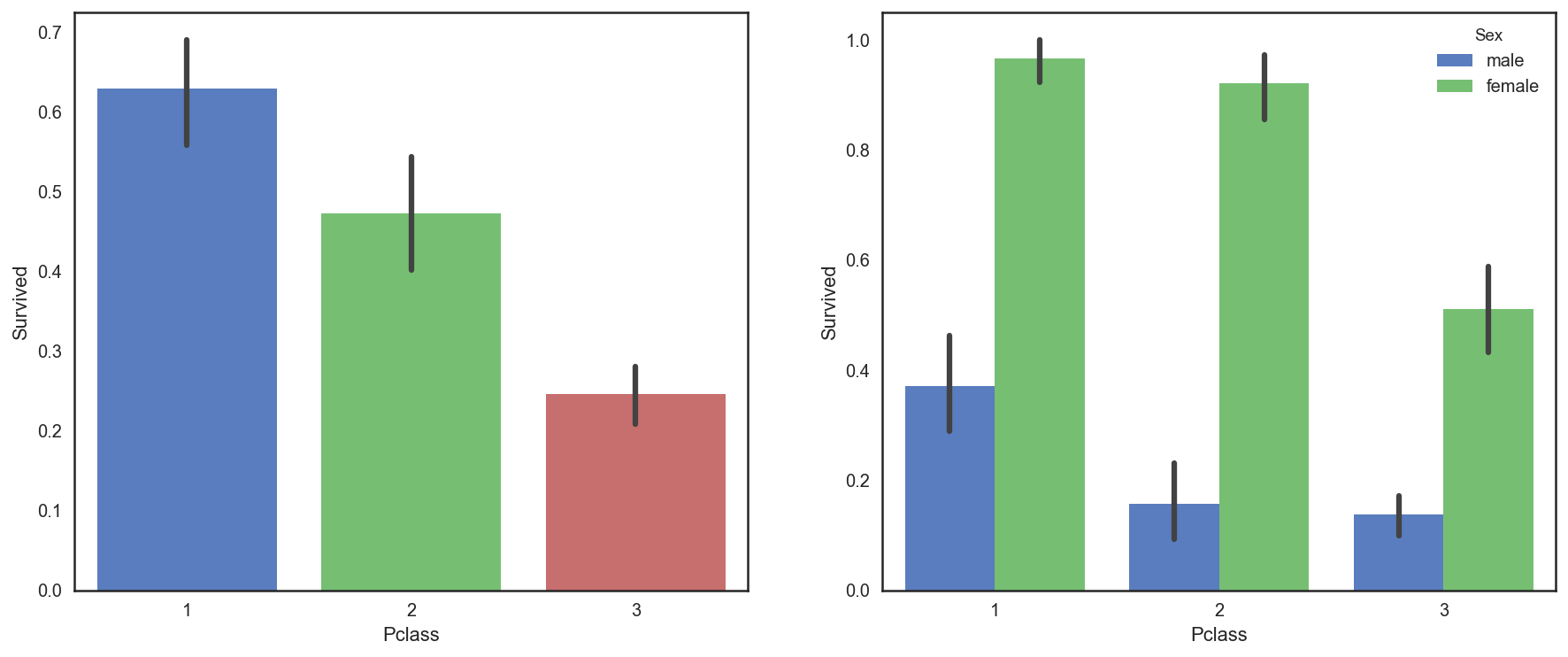- The passenger survival is not the same in the 3 classes. First class passengers have more chance to survive than second class and third class passengers.

#### Embarked¶

Definition : Port of Embarkation

• C = Cherbourg
• Q = Queenstown
• S = Southampton
In :
all_data["Embarked"].isnull().sum()

Out:
2

Note : Since we have two missing value , I decided to fill it with the most fequent value of "Embarked"(S)

In :
#Fill Embarked nan values of dataset set with 'S' most frequent value
all_data["Embarked"] = all_data["Embarked"].fillna("S")

In :
# Explore Embarked vs Survived
g = sns.barplot(x="Embarked", y="Survived",  data=train)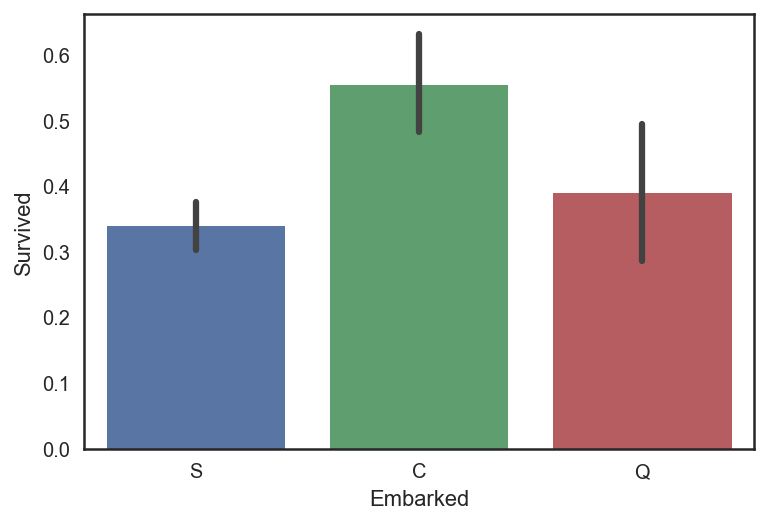- It seems that passenger coming from Cherbourg (C) have more chance to survive.

- My hypothesis is that the proportion of first class passengers is higher for those who came from Cherbourg than Queenstown (Q), Southampton (S).

- Let's see the Pclass distribution vs Embarked
In :
# Explore Pclass vs Embarked
g = sns.factorplot("Pclass", col="Embarked",  data=train,
size=6, kind="count", palette="muted")
g.despine(left=True)
g = g.set_ylabels("Count")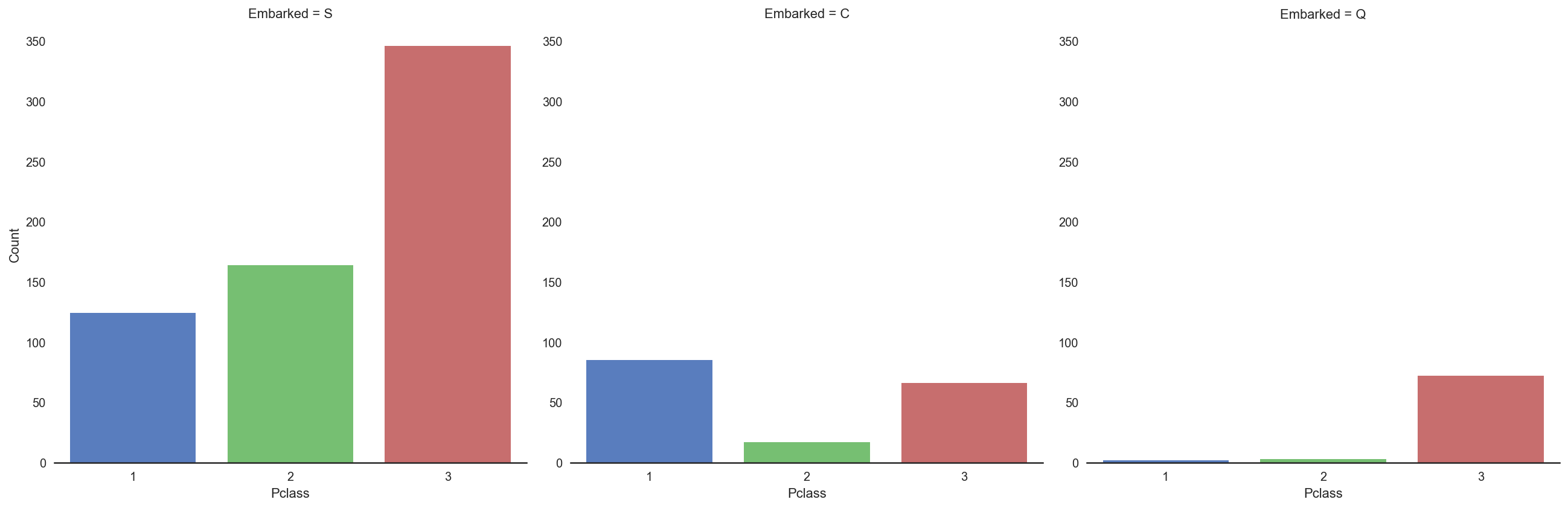- Indeed, the third class is the most frequent for passenger coming from Southampton (S) and Queenstown (Q), whereas Cherbourg passengers are mostly in first class which have the highest survival rate.

- I think that first class passengers were prioritised during the evacuation.

## 4. Filling missing Values¶

### 4.1 Age¶

In :
all_data["Age"].isnull().sum()

Out:
256

Note :

- As we see, Age column contains 256 missing values in the whole dataset.

- Since there is subpopulations that have more chance to survive (children for example), it is preferable to keep the age feature and to impute the missing values.

- To adress this problem, I looked at the most correlated features with Age (Sex, Parch , Pclass and SibSP).
In :
# Explore Age vs Sex, Parch , Pclass and SibSP
plt.figure(figsize=(20,10))

ax1 = plt.subplot(2,2,1)
sns.boxplot(y="Age",x="Sex",data=all_data, ax=ax1)

ax2 = plt.subplot(2,2,2)
sns.boxplot(y="Age",x="Sex",hue="Pclass", data=all_data, ax=ax2)

ax3 = plt.subplot(2,2,3)
sns.boxplot(y="Age",x="Parch", data=all_data, ax=ax3)

ax4 = plt.subplot(2,2,4)
sns.boxplot(y="Age",x="SibSp", data=all_data, ax=ax4)

plt.show()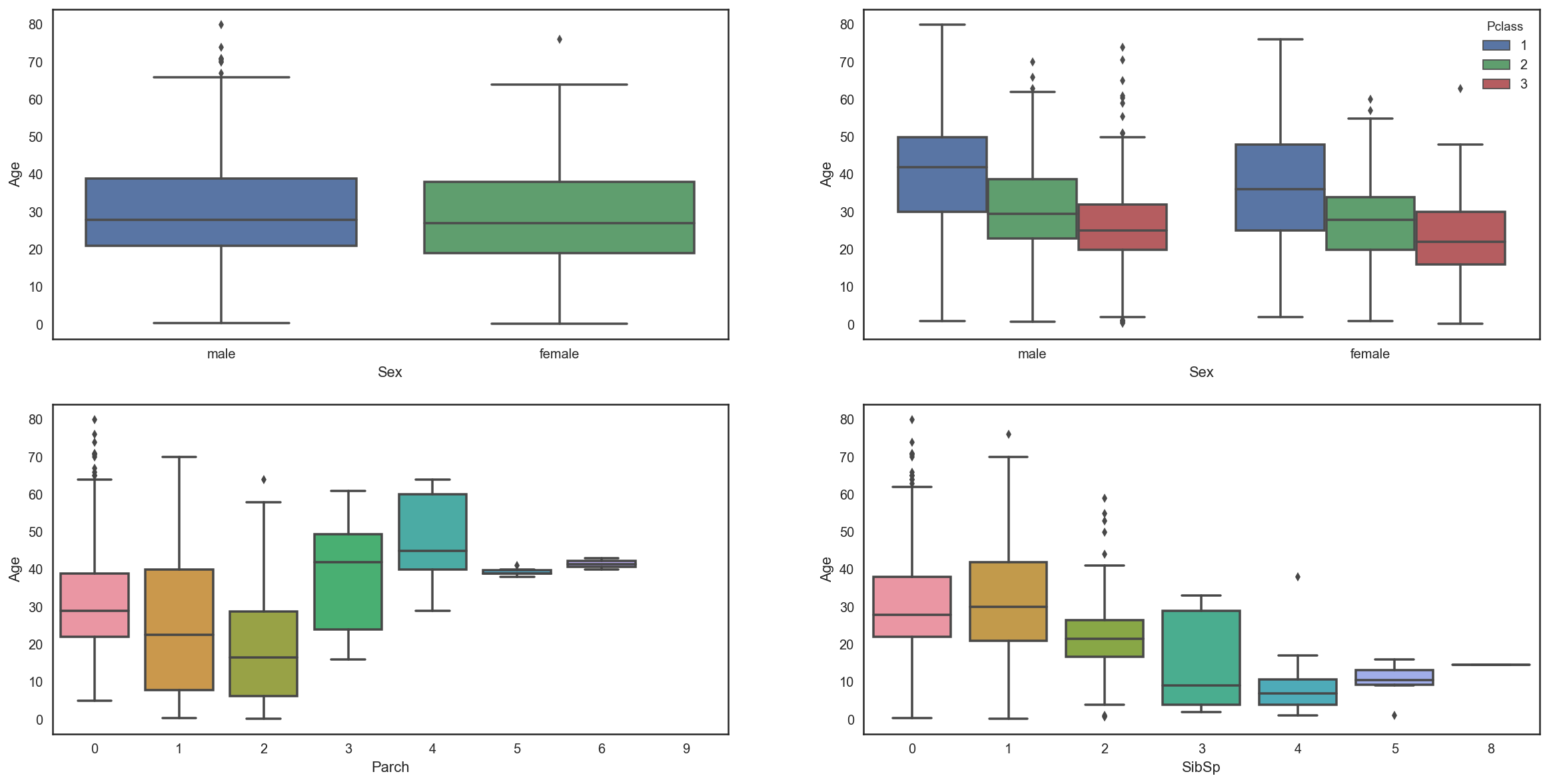- Age distribution seems to be the same in Male and Female subpopulations, so Sex is not informative to predict Age.

- However, 1st class passengers are older than 2nd class passengers who are also older than 3rd class passengers.

- Moreover, the more a passenger has parents/children the older he is and the more a passenger has siblings/spouses the younger he is.
In :
# convert Sex into categorical value 0 for male and 1 for female
all_data["Sex"] = all_data["Sex"].map({"male": 0, "female":1})

In :
numeric = ["Sex","SibSp","Parch","Pclass","Fare","Age"]
corr = all_data[numeric].corr()
with sns.axes_style("white"):
ax = sns.heatmap(corr, mask = mask, annot=True, fmt = ".2f", cmap = "YlGnBu")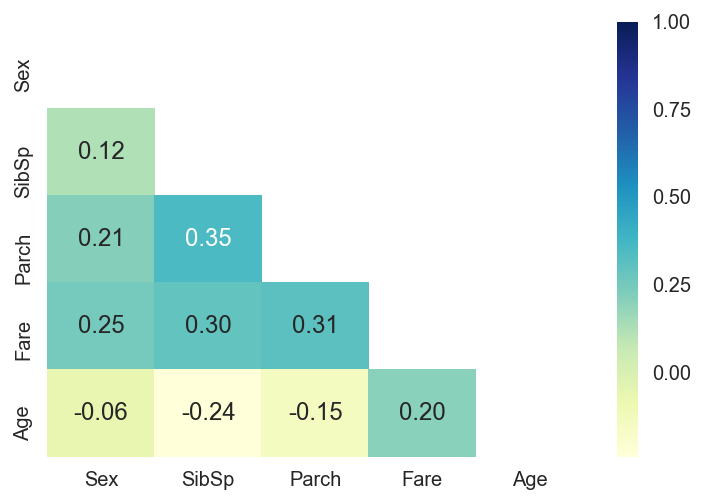- The correlation map confirms that Age is negatively correlated with Pclass and SibSp.

- I decided to use SibSP and Pclass in order to impute the missing ages.

- My plan is to fill Age with the median age of similar rows according to Pclass and SibSp.
In :
# fill Age with the median age of similar rows according to Pclass and SibSp

age_nan = list(all_data["Age"][all_data["Age"].isnull()].index)

for i in age_nan:
age_median = all_data["Age"].median()
age_pred = all_data["Age"][((all_data['SibSp'] == all_data.iloc[i]['SibSp']) & (all_data['Pclass'] == all_data.iloc[i]['Pclass']))].median()
if not np.isnan(age_pred) :
all_data['Age'].iloc[i] = age_pred
else :
all_data['Age'].iloc[i] = age_median


## 5. Feature engineering¶

### 5.1 Name/Title¶

In :
all_data["Name"].head()

Out:
0                              Braund, Mr. Owen Harris
1    Cumings, Mrs. John Bradley (Florence Briggs Th...
2                               Heikkinen, Miss. Laina
3         Futrell